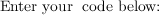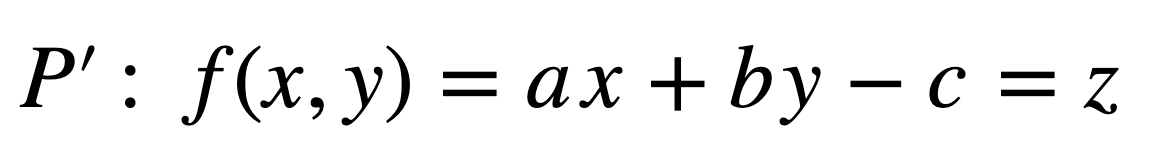1596337740

# Weighted Least Squares

Weighted Least Square is an estimate used in regression situations where the error terms are heteroscedastic or has non constant variance.

To get a better understanding about Weighted Least Squares, lets first see what Ordinary Least Square is and how it differs from Weighted Least Square.

#### What is Ordinary Least Square(OLS)?

In a simple linear regression model of the form,whereis the independent variableis the independent variableandare the regression coefficientsis the random error or the residual.

The goal is to find a line that best fits the relationship between the outcome variableand the input variable. With OLS, the linear regression model finds the line through these points such that the sum of the squares of the difference between the actual and predicted values is minimum.

i.e., to findandsuch thatis minimum.

In such linear regression models, the OLS assumes that the error terms or the residuals (the difference between actual and predicted values) are normally distributed with mean zero and constant variance. This constant variance condition is called homoscedasticity.

If this assumption of homoscedasticity does not hold, the various inferences made with this model might not be true.

To check for constant variance across allvalues along the regression line, a simple plot of the residuals and the fitted outcome values and the histogram of residuals such as below can be used.

In an ideal case with normally distributed error terms with mean zero and constant variance , the plots should look like this.From the above plots its clearly seen that the error terms are evenly distributed on both sides of the reference zero line proving that they are normally distributed with mean=0 and has constant variance.

The histogram of the residuals also seems to have datapoints symmetric on both sides proving the normality assumption.

In some cases, the variance of the error terms might be heteroscedastic, i.e., there might be changes in the variance of the error terms with increase/decrease in predictor variable.

In those cases of non-constant variance Weighted Least Squares (WLS) can be used as a measure to estimate the outcomes of a linear regression model.

Now let’s see in detail about WLS and how it differs from OLS.

#python

## Buddha Community1604056260

## In-depth analysis of the regularized least-squares algorithm

This article will introduce key concepts about Regularized Loss Minimization (RLM) and Empirical Risk Minimization (ERM), and it’ll walk you through the implementation of the least-squares algorithm using MATLAB. The models obtained using RLM and ERM will then be compared and discussed against each other.

We’ll use a polynomial curve-fitting problem to predict the best polynomial for this data. The least-squares algorithm will be implemented step-by-step using MATLAB.

By the end of this post, you’ll understand the least-squares algorithm and be aware of the advantages and downsides of RLM and ERM. Additionally, we’ll discuss some important concepts about overfitting and underfitting.

## Dataset

We’ll use a simple one input dataset with N = 100 data points. This dataset was originally proposed by Dr. Ruth Urner on one of her assignments for a machine learning course. In the repository below, you’ll find two TXT files: dataset1_inputs.txt and dataset1_outputs.txt.

These files contain the input and output vectors. Using MATLAB, we’ll plot these data points in a chart. On MATLAB, I imported them in Home > Import Data. Then, I created the flowing script for plotting the data points.

#data-science #programming #polynomial-regression #least-squares #machine-learning1598299980

## How to find the least squares plane from a cloud of point using Excel, Numbers etc…

### In this article I will attempt to explain how we can use a spreadsheet to to find the least squares plane from a cloud of point in a 3D space. And hopefully you will have a better understanding of this method.

First of all let’s consider our set of point. Each point has three coordinates, x, y and z where x, y, z are three reals. You can see the set of point use in this exemple in the following picture.

Now that we have our points we can start digging into the math. Let me remind you what a plan is mathematicaly speaking. A plan is the result of a linear fonction of two variables, these fancy words simply means that for a couple of two numbers _x _and y, the function gives you a third number z, thus the link between x, y and z is linear, meaning the operations done on x and y are only multiplication with a real or addition with a real. Finally we can write this equation for a plan P’ :Any given couple of _x _and y has a corresponding z value, we can use our x and y values in this equation to express the gap between the z value of our point and the z value it should have if it was part of this “ideal” plan. We will call this new function g for gap.

#numbers #mathematics #excel #least-squares #spreadsheets #cloud1596337740

## Weighted Least Squares

Weighted Least Square is an estimate used in regression situations where the error terms are heteroscedastic or has non constant variance.

To get a better understanding about Weighted Least Squares, lets first see what Ordinary Least Square is and how it differs from Weighted Least Square.

#### What is Ordinary Least Square(OLS)?

In a simple linear regression model of the form,whereis the independent variableis the independent variableandare the regression coefficientsis the random error or the residual.

The goal is to find a line that best fits the relationship between the outcome variableand the input variable. With OLS, the linear regression model finds the line through these points such that the sum of the squares of the difference between the actual and predicted values is minimum.

i.e., to findandsuch thatis minimum.

In such linear regression models, the OLS assumes that the error terms or the residuals (the difference between actual and predicted values) are normally distributed with mean zero and constant variance. This constant variance condition is called homoscedasticity.

If this assumption of homoscedasticity does not hold, the various inferences made with this model might not be true.

To check for constant variance across allvalues along the regression line, a simple plot of the residuals and the fitted outcome values and the histogram of residuals such as below can be used.

In an ideal case with normally distributed error terms with mean zero and constant variance , the plots should look like this.From the above plots its clearly seen that the error terms are evenly distributed on both sides of the reference zero line proving that they are normally distributed with mean=0 and has constant variance.

The histogram of the residuals also seems to have datapoints symmetric on both sides proving the normality assumption.

In some cases, the variance of the error terms might be heteroscedastic, i.e., there might be changes in the variance of the error terms with increase/decrease in predictor variable.

In those cases of non-constant variance Weighted Least Squares (WLS) can be used as a measure to estimate the outcomes of a linear regression model.

Now let’s see in detail about WLS and how it differs from OLS.

#python1624114920

## Self hosted FLOSS fitness/workout, nutrition and weight tracker written with Django

### wger

wger (ˈvɛɡɐ) Workout Manager is a free, open source web application that help you manage your personal workouts, weight and diet plans and can also be used as a simple gym management utility. It offers a REST API as well, for easy integration with other projects and tools.### Mobile app### Installation

These are the basic steps to install and run the application locally on a Linux

system. There are more detailed instructions, other deployment options as well

in your code repository in the docs folder.

Please consult the commands’ help for further information and available

parameters.

#### Production

If you want to host your own instance, take a look at the provided docker

https://github.com/wger-project/docker

#django #self hosted floss fitness/workout #weight tracker written #self hosted floss fitness/workout, nutrition and weight tracker written with django #floss #nutrition

1605870015

## Healthy Weight Gain Products, How To Gain Weight By Ayurveda

Friends if you take our parivartan ayurveda weight gain capsule. You can gain weight in 15 days. If you take a daily protein diet, then parivartan ayurveda weight gain capsules you will gain 5kg in a month.

Friends, if you also want to build a good body and want to increase your weight. You can call us anytime from Monday to Saturday from 9.30 am to 6 pm and book your weight gain product. We assure you that we will give you 100% complete Ayurvedic products, which will help you in building your body.

Friends, if you are thin, it is a bit difficult to live in a place where all the population is fit and healthy and you are the only underweight person. People start throwing their lines like they pass comments like you don’t eat anything? Why are you so thin? Such situations are shameful. And at that time, the first question that keeps us in mind is how do we increase our weight?

Our dear friends, if you are slim and desire to increase your weight, then your Parivartan Ayurveda Company offers you 100% safe and Ayurvedic capsules for weight gain within a month. If you want to increase your weight quickly and safely, you can call us now. Our number is + 91-9250050227, + 91-9250050228. Our team is available to serve you from Monday to Saturday from 9.30 am to 6 pm.

Friends, if you use the products of Parivartan Ayurveda, you will 100% definitely benefit from our products, which will be very beneficial for your health. Increase your weight safely, give us a chance to serve once. If you use Parivartan Ayurveda products, we assure you that you will get 100% Piero Ayurvedic products.

#how to gain weight